Test: Surface Tension

# Test: Surface Tension

Test Description

## 10 Questions MCQ Test Fluid Mechanics | Test: Surface Tension

Test: Surface Tension for Civil Engineering (CE) 2022 is part of Fluid Mechanics preparation. The Test: Surface Tension questions and answers have been prepared according to the Civil Engineering (CE) exam syllabus.The Test: Surface Tension MCQs are made for Civil Engineering (CE) 2022 Exam. Find important definitions, questions, notes, meanings, examples, exercises, MCQs and online tests for Test: Surface Tension below.
Solutions of Test: Surface Tension questions in English are available as part of our Fluid Mechanics for Civil Engineering (CE) & Test: Surface Tension solutions in Hindi for Fluid Mechanics course. Download more important topics, notes, lectures and mock test series for Civil Engineering (CE) Exam by signing up for free. Attempt Test: Surface Tension | 10 questions in 25 minutes | Mock test for Civil Engineering (CE) preparation | Free important questions MCQ to study Fluid Mechanics for Civil Engineering (CE) Exam | Download free PDF with solutions
 1 Crore+ students have signed up on EduRev. Have you?
Test: Surface Tension - Question 1

### Which of the following contribute to the reason behind the origin of surface tension?

Detailed Solution for Test: Surface Tension - Question 1

Explanation: The molecules on the surface of a liquid experience cohesive forces due to surrounding liquid molecules acting downward and adhesive forces due to surrounding gaseous molecules acting upwards. Surface tension orginates due to this unbalanced force on the surface molecules.

Test: Surface Tension - Question 2

### A soap film is trapped between a frame and a wire of length 10 cm as shown.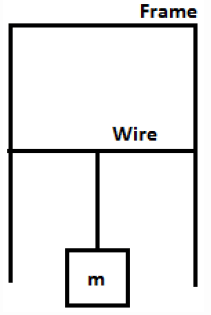If the surface tension is given as 0.0049 N/m, what will be the value of m (in mg) such that the wire remains in equilibrium?

Detailed Solution for Test: Surface Tension - Question 2

Explanation: For the wire to be in equilibrium, Force exerted by the film on the wire due to surface tension (acting upwards) must be equal to the downward force due to the weight of the wire (acting downwards). If σ=surface tension, l=length of the wire
2σl = mg
Substituting all the values, m = 2σl/g = 2 * 0.0049 * 0.01 ⁄ 9.81 = 99.9mg.

Test: Surface Tension - Question 3

### What will be the diameter (in mm) of a water droplet, the pressure inside which is 0.05 N/cm2 greater than the outside pressure? (Take surface tension as 0.075 N/m)

Detailed Solution for Test: Surface Tension - Question 3

Explanation: p = 4σ/d
where p = pressure difference between the liquid droplet and the surrounding medium, σ = surface tension and d = diameter of the droplet. Substituting all the values,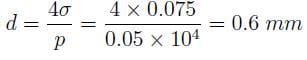Test: Surface Tension - Question 4

A soap bubble of d mm diameter is observed inside a bucket of water. If the pressure inside the bubble is 0.075 N/cm2, what will be the value of d? (Take surface tension as 0.075 N/m)

Detailed Solution for Test: Surface Tension - Question 4

Explanation: p = 8σ/d
where p = pressure difference between the bubble and the surrounding medium, σ = surface tension and d = diameter of the bubble. Substituting all the values,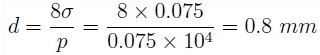Test: Surface Tension - Question 5

Find the pressure difference of a liquid jet of 5 cm diameter. (Take surface tension as 0.075 N/m)

Detailed Solution for Test: Surface Tension - Question 5

p = σ/d
where p = pressure difference between the bubble and the surrounding medium, σ = surface tension and d = diameter of the bubble. Substituting all the values,
p = 0.075 / 5 * 10-2 = 1.5 N/m2.

Test: Surface Tension - Question 6

The rise in the level of a liquid in a tube is h. What will be the rise in the level if the same amount of liquid is poured into a tube of half the diameter.

Detailed Solution for Test: Surface Tension - Question 6

Explanation: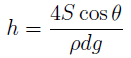where h = rise in liquid height in the tube, S = surface tension, θ = the angle of contact, d = diameter of the tube, ρ = density of liquid and g = acceleration due to gravity. All other factors remaining constant, h α d. Thus, if d is halved, h will be doubled.

Test: Surface Tension - Question 7

The ratio of the surface tension S and density ρ of liquid 1 and 2 are 1:2 and 1:4 respectively. Equal amount of the two liquids is poured into two identical tubes. what will be the ratio of the rise in the liquid level in the two tubes? (Assume the angle of contact to be same)

Detailed Solution for Test: Surface Tension - Question 7

Explanation:where h = rise in liquid height in the tube, S = surface tension, θ = the angle of contact, d = diameter of the tube, ρ = density of liquid and g = acceleration due to gravity.
Given, S1 / ρ1 = 1 : 2 and S2 / ρ2 = 1 : 4.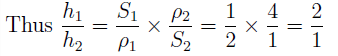Test: Surface Tension - Question 8

The rise in the level of a liquid in a tube is h. If half the amount is poured outside, what will be the new rise in liquid level?

Detailed Solution for Test: Surface Tension - Question 8

Explanation: The rise in liquid level for a liquid is independent of the amount of liquid present in the tube. Since, same tube is used and same liquid is considered, the rise in the liquid level will remain the same.

Test: Surface Tension - Question 9

If a glass tube of 10 mm diameter is immersed in water, what will be the rise or fall in capillary?
(Take surface tension = 0.075 N/m, g = 10 m/s2 and angle of contact = 0)

Detailed Solution for Test: Surface Tension - Question 9

Explanation: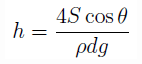where h = rise in liquid height in the tube, S = surface tension, θ = the angle of contact, d = diameter of the tube, ρ = density of liquid and g = acceleration due to gravity. Substituting all the values,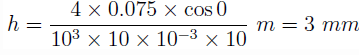Test: Surface Tension - Question 10

A water drop of diameter 1 cm breaks into 1000 similar droplets of same diameter. What will be the gain or loss in the surface energy? (Take surface tension as 0.075 N/m)

Detailed Solution for Test: Surface Tension - Question 10

Explanation: According to the Principle of Conservation of mass, M = 1000 * m, where M = mass of the big drop, m = mass of each droplet. Assuming density to be constant, D3 = 1000 * d3, i.e. D = 10d, where D = diameter of big drop, d = diameter of a droplet.
Change in surface energy = Surface tension * Change in surface area = 0:075*(1000 * πd2– πD2) = 0:075 * (10 * πD2 – πD2) = 0:075 * 9π * (10-2)2 = 0:212 mJ Since, the change is positive, there will be a gain in the surface energy.

## Fluid Mechanics

43 videos|72 docs|72 tests
 Use Code STAYHOME200 and get INR 200 additional OFF Use Coupon Code
Information about Test: Surface Tension Page
In this test you can find the Exam questions for Test: Surface Tension solved & explained in the simplest way possible. Besides giving Questions and answers for Test: Surface Tension, EduRev gives you an ample number of Online tests for practice

## Fluid Mechanics

43 videos|72 docs|72 tests